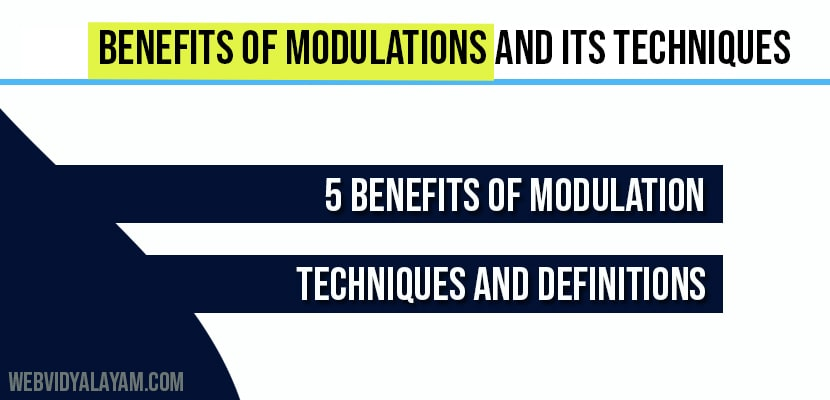# Benefits of modulations and its Techniques

Modulation allows you to transmit information using radio signals and benefits of modulation includes height of the antenna is reduced, it reduces the interference among the signals, mixing of signals is reduced, multiplexing of signals is possible, long distance communication is possible.

## 1 .Height of the Antenna is Reduced:

We know that there are transmitting and receiving antennas for transmission and reception of signals. The condition for efficient transmission and reception is that the antenna height must be the  multiples of λ/4.

λ=c/f

λ= wavelength of the frequency

c=speed of light=3*108m/s

This implies that the antenna height and wavelength are inversely proportional. In practical by using the above formula for antenna height it is impossible to construct the antenna with the obtained output height therefore by  using some modulation height of the antenna is reduced.

## 2. Reduces the Interference Among the Signals:

Interference can b reduced among the signals by modulating each baseband signal with different frequencies of carrier signal so that each baseband signal has different frequency slots so that many signals can be transmitted simultaneously.

## 3. Mixing of the Signals is Reduced:

If the baseband signals are transmitted without modulation all the signals gets mixed as they have same frequency range. With modulation this problem is solved.

## 4. Multiplexing of the Signals is Possible:

With modulation many signals can be transmitted over a single channel receiver receives those signals and separates them using band pass filter.

## 5. Long Distance Communication is Possible:

Baseband signals have low frequency range these low frequency signals experiences attenuation when they are transmitted to longer distances  therefore they travels only short distances. Modulation increases the frequency of the baseband signals and allows to travel  longer distances

## Definitions:

### Carrier Signal:

carrier signal is an  alternating empty signal with higher frequency used for carrying the information.

### Modulating Signal:

The signal that is to be modulated is called modulating signal

### Modulated Wave:

The signal that is obtained after modulation process is known as modulated wave.

### Multiplexing:

Multiplexing is the process of transmitting multiple signals over a single communication channel.

## Questions:

1. What is modulation? Why do we need modulation?

2. Benefits of modulation or advantages of modulation?

3. Define the terms signal, carrier signal, modulating signal, modulated wave?

4. Define the term multiplexing.

## Modulation Techniques:

Two types of modulation techniques and they are Analog modulation and Digital modulation. Firstly we discuss Analog modulation techniques later we move on to Digital modulation techniques.

## Analog modulation techniques:

1. Amplitude Modulation

2. Frequency Modulation

3. Phase modulation